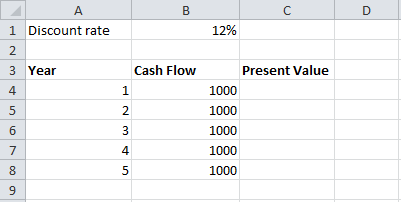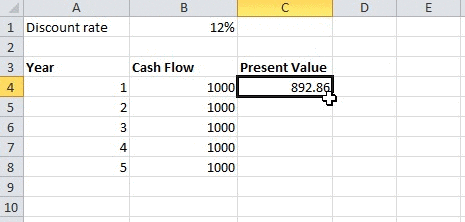# Financial Modelling: Writing Formulas in Excel

In have already looked at the excel keyboard shortcuts that you must know to become productive with excel. The next thing we will look at is how to write effective formulas.

This is an important topic and I believe the quality of your financial model will depend on how well you write the formulas. There are three reasons why it is very important to think and plan about how you write each formula:

1. Your financial model is probably going to be used by others in your organizations and they should be able to make use of what you’ve created.
2. You will most likely be using the financial model for a long period and when you use it, you may have to tweak it. You shouldn’t have to make major changes to the formulas every time you want to extend the model. For example, if your model contained financial projects upto 2018, and the next year you want to extend it upto 2020, it should simply be a matter of copying and pasting the formulas to the new cells/rows rather than having to make changes to the formula.
3. The formula should be simple and short so that you yourself can make sense of it when you try to read it after a long gap.

Let’s take a simple example of calculating the present value of a series of cash flows. Let’s say that you are making an investment that will pay you \$1,000 at the end of each year for the next five years. If you had placed the money in a bank deposit, you would have received an interest of 12% p.a. Let’s say that this is the interest that your investment also pays. Based on this information, we can calculate the value of this investment today. Let’s see how.

The below screenshot shows the information that we have:We can calculate the value of this investment by discounting the cash flows with the discount rate. Alternatively we can also calculate it using Excel’s built-in PV function. The following screenshot shows the formula using the first method:Let’s dissect the formula to understand what we’ve done. I press CTRL + ` to view all formulas in the spreadsheet as shown below: